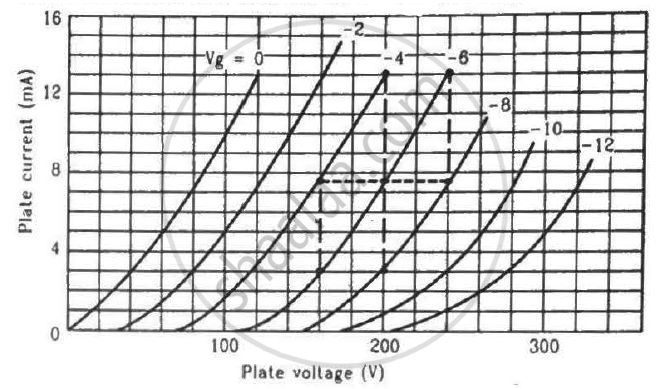Department of Pre-University Education, KarnatakaPUC Karnataka Science Class 12
Share

# Find the Values of Rp, µ and Gm of a Triode Operating at Plate Voltage 200 V and Grid Voltage −6. the Plate Characteristics Are Shown in the Figure. - Physics

ConceptSemiconductor Diode

#### Question

Find the values of rp, µ and gm of a triode operating at plate voltage 200 V and grid voltage −6. The plate characteristics are shown in the figure.#### Solution

Dynamic plate resistance, r_p=((deltaV_p)/(deltaI_p)), at constant grid voltage

We need to find the slope of the graph for a particular value of grid voltage, i.e. Vg = −6 V.

Consider two points for the plot of Vg = −6 V:-

$r_p = \frac{(240 - 160) V}{(13 - 3) \times {10}^{- 3} A}$

$r_p = \frac{80}{10} \times {10}^3 \Omega$

$r_p = 8 K\Omega$

$g_m = \left( \frac{\delta I_p}{\delta V_g} \right)_{V_P = \text{constant } (200 V)}$

Consider two points on the 200 V line:-

$g_m = \frac{(13 - 3) \times {10}^{- 3}}{[( - 4) - ( - 8)]}A$

$g_m = \frac{10 \times {10}^{- 3}}{4}=2.5\text{ mili mho}$

Amplification factor,

$\mu = - \left( \frac{∆ V_P}{∆ V_G} \right)_{i_P =\text{ constant}}$

$\mu = - \frac{100 - 180}{- 6 - ( - 10)}$

$\mu = \frac{80}{4} = 20$

Is there an error in this question or solution?

#### Video TutorialsVIEW ALL 

Solution Find the Values of Rp, µ and Gm of a Triode Operating at Plate Voltage 200 V and Grid Voltage −6. the Plate Characteristics Are Shown in the Figure. Concept: Semiconductor Diode.
S